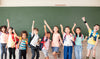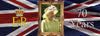3D shapes or three-dimensional shapes are not flat. They can be described using lots of different mathematical vocabulary. See how many of the 3D shapes below you recognise. Try to use the mathematical vocabulary to describe each of the shapes.

## Important 3D Shape Vocabulary

The side of a 3D shape is called the face. 3D shape faces are flat.

The edges of a 3D shape are where the faces meet.

The corners of a 3D shape are called vertices. Vertex is the singular of vertices

A regular 3D shape’s faces are all equal. A cube is an example of a regular 3D shape. It has six square faces that are all the same size.

An irregular 3D shape has faces of differing shapes and sizes. A cylinder is an example of an irregular 3D shape. It has three faces, two are circles and one is a rectangle.

### Polyhedron

A polyhedron is a 3D shape made up of a collection of flat polygons. Polyhedrons can have any number of faces. Triangular prisms and cubes are examples of polyhedrons.

If you want to know more about 2D shapes, including polygons, make sure you read our 2D Shape Blog.

## 3D shape names and facts

Look below to learn all about the properties of 3D shapes in more detail.

## Sphere

How many of these sphere facts do you know?

• A sphere is a three-dimensional shape.
• A sphere has 1 curved face.
• A sphere has no vertices.
• A sphere has no edges.
• A sphere is symmetrical.
• A sphere is not a polyhedron.

## Hemisphere

How many of these hemisphere facts do you know?

• A hemisphere is a three-dimensional shape.
• A hemisphere has 2 faces. 1 curved face and 1 flat circular face.
• A hemisphere has no vertices.
• A hemisphere has 1 edge.
• A hemisphere is half of a sphere.
• A hemisphere is not a polyhedron.

## Cube

How many of these cube facts do you know?

• A cube is a three-dimensional shape.
• A cube has 6 flat faces.
• A cube's faces are all squares. They are all the same size and shape.
• A cube has 8 vertices.
• A cube has 12 edges.
• A cube is a polyhedron.

## Cuboid or a rectangular prism

How many of these cuboid facts do you know?

• A cuboid is a three-dimensional shape.
• A cuboid has 6 flat faces.
• A cuboid's faces are all rectangles.
• A cuboid has 8 vertices.
• A cuboid has 12 edges.
• A cuboid doesn’t have to have regular faces like a cube.
• A cuboid is a polyhedron.

## Cone

How many of these cone facts do you know?

• A cone is a three-dimensional shape.
• A cone has 2 faces. One is a flat circular face. The other is a curved face.
• A cone has 1 apex. (The pointed tip of a cone is called the apex.)
• A cone has 1 edge.
• The flat circular face of a cone is the base.
• A cone is not a polyhedron.

## Cylinder

How many of these cylinder facts do you know?

• A cylinder is a three-dimensional shape.
• A cylinder has 3 faces. Two of the faces are flat circular faces, one is a curved rectangular face.
• A cylinder has 0 vertices.
• A cylinder has 2 edges.
• A cylinder is not a polyhedron.

Download this free 3D shape nets worksheet to find out for yourself one of the faces of a cylinder is a curved rectangle.

## Triangular prism

How many of these triangular prism facts do you know?

• A triangular prism is a three-dimensional shape.
• A triangular prism has 5 faces. Two of the faces are flat triangular faces, three are flat rectangular faces.
• A triangular prism has 6 vertices.
• A triangular prism has 9 edges.
• A triangular prism is a polyhedron.

## Pentagonal prism

How many of these pentagonal prism facts do you know?

• A pentagonal prism is a three-dimensional shape.
• A pentagonal prism has 7 faces. Two of the faces are flat pentagonal faces, five are flat rectangular faces.
• A pentagonal prism has 10 vertices.
• A pentagonal prism has 15 edges.
• A pentagonal prism is a polyhedron.

## Hexagonal prism

How many of these hexagonal prism facts do you know?

• A hexagonal prism is a three-dimensional shape.
• A hexagonal prism has 8 faces. Two of the faces are flat hexagonal faces, six are flat rectangular faces.
• A hexagonal prism has 12 vertices.
• A hexagonal prism has 18 edges.
• A hexagonal prism is a polyhedron.

## Triangular based pyramid or Tetrahedron

How many of these triangular based pyramid facts do you know?

• A triangular based pyramid is a three-dimensional shape.
• A triangular based pyramid has 4 faces. All of the faces are flat triangular faces.
• A triangular based pyramid has 4 vertices.
• A triangular based pyramid has 6 edges.
• A triangular based pyramid is a polyhedron.

## Square based pyramid

How many of these square based pyramid facts do you know?

• A square based pyramid is a three-dimensional shape.
• A square based pyramid has 5 faces. Four of the faces are flat triangular faces, one of the faces is a square.
• A square based pyramid has 5 vertices.
• A square based pyramid has 8 edges.
• A square based pyramid is a polyhedron.

## Rectangular based pyramid

How many of these rectangular based pyramid facts do you know?

• A rectangular based pyramid is a three-dimensional shape.
• A rectangular based pyramid has 5 faces. Four of the faces are flat triangular faces, one of the faces is a rectangle.
• A rectangular based pyramid has 5 vertices.
• A rectangular based pyramid has 8 edges.
• A rectangular based pyramid is a polyhedron.

If you want to know more about shapes make sure you read our blogs about 2D Shape Blog, shape angles, area and perimeter and symmetry

## When are 3D shapes taught in the Maths National Curriculum?

3D shapes are taught as part of the geometry strands of the maths curriculum. This strand is geometry - properties of shapes

3D shapes are first taught in year 1 when children:

In Year 2 children:

In Year 3 children:

In Year 5 children:

In Year 6 children:

### Related Posts##### Top ways to embed RE in your classroom
Many teachers would agree that some subjects are easier to embed in the classroom than others. We have put together some##### Fun ways to engage children in reading
Have you ever struggled with a group of reluctant readers? We understand the importance of reading in a child's developm##### Queen Elizabeth II Facts for Kids
Queen Elizabeth II is about to celebrate her Platinum Jubilee, but how much do you know about our sovereign? Read these